# 线段树从零开始

By 岩之痕

 题目一： 10000个正整数，编号1到10000，用A,A,A表示。 修改：无 统计：1.编号从L到R的所有数之和为多少？ 其中1<= L <= R <= 10000.

 题目二： 10000个正整数，编号从1到10000，用A,A,A表示。 修改：1.将第L个数增加C （1 <= L <= 10000） 统计：1.编号从L到R的所有数之和为多少？ 其中1<= L <= R <= 10000.

 方法一 方法二 A[L]+=C 修改1个元素 修改R-L+1个元素 求和A[L..R] 计算R-L+1个元素之和 计算两个元素之差

A[L]+=C 需要修改log2(10000) 个元素

log2(10000) < 14 所以相对来说线段树的修改和求和都比较快。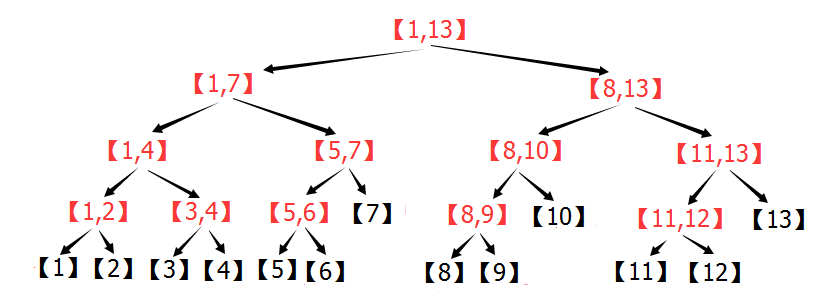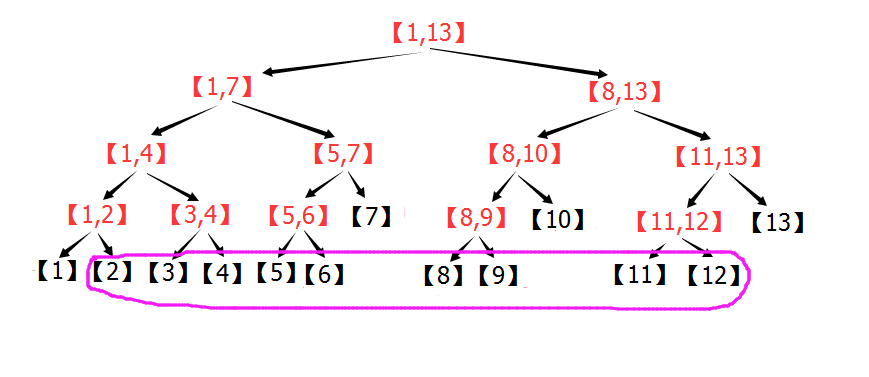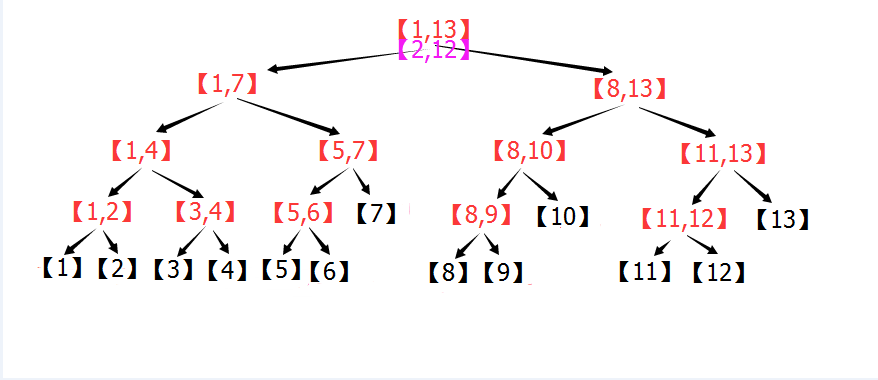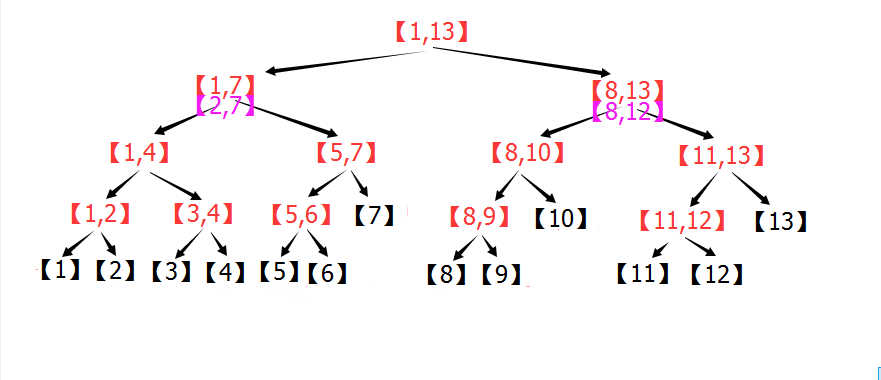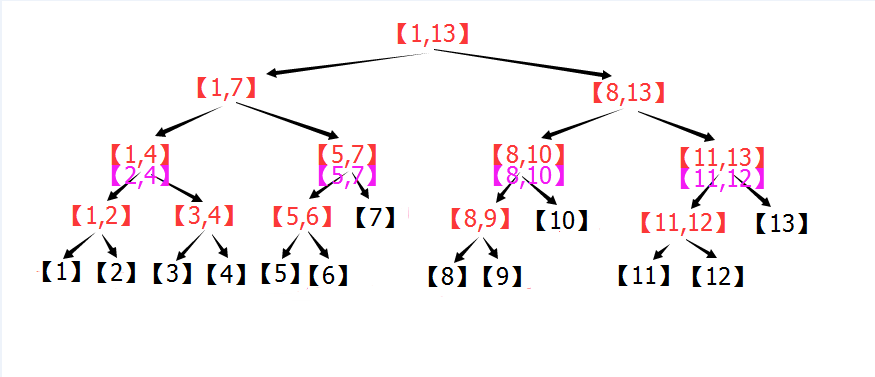[5,7]处于节点[5,7]的位置，所以不用继续分解，[2,4]处于区间[1,4]的位置，所以继续分解成和[3,4]。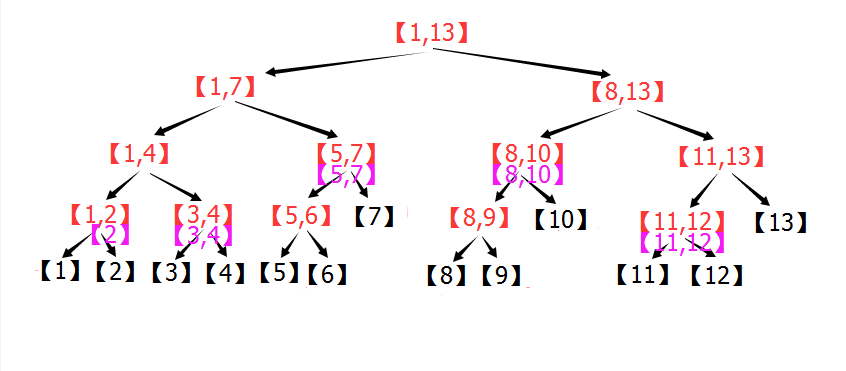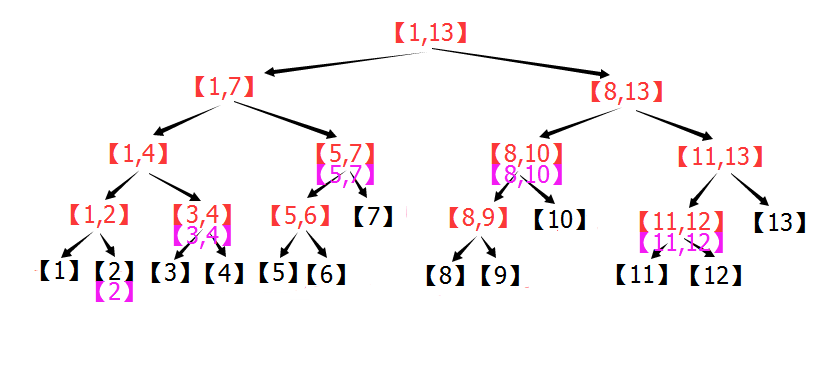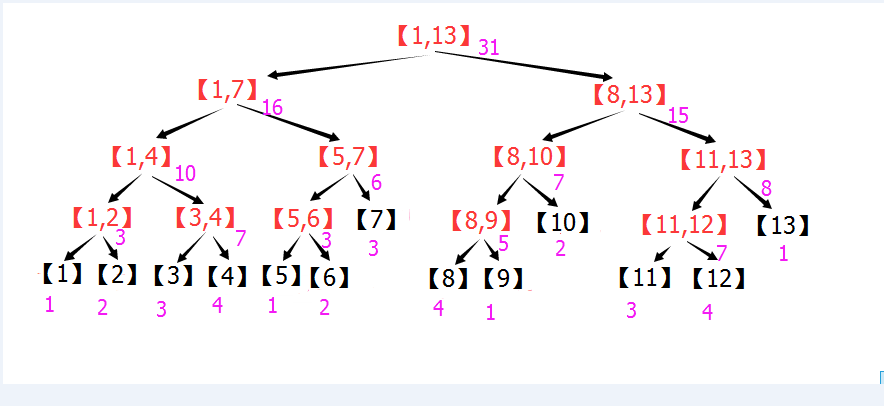[2,12]= + [3,4] + [5,7] + [8,10] + [11,12]
29  = 2 + 7 + 6 + 7 + 7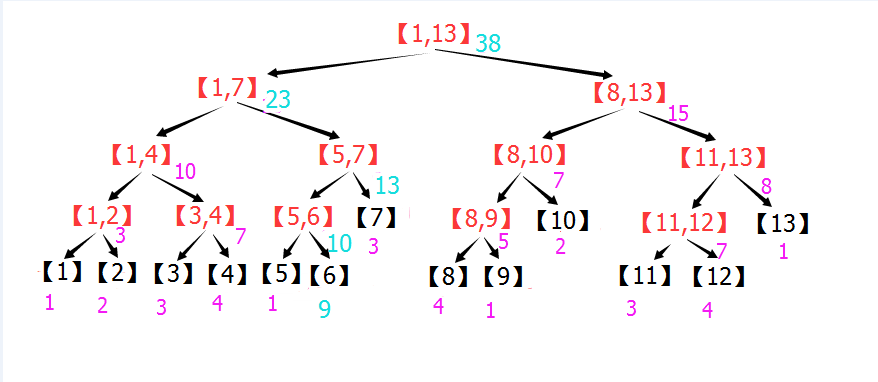(0)定义：

[cpp]  view plain  copy

1. #define maxn 100007  //元素总个数
2. int Sum[maxn<<2];//Sum求和，开四倍空间
3. int A[maxn],n;//存原数组下标[1，n]

(1)建树：

[cpp]  view plain  copy

1. //PushUp函数更新节点信息，这里是求和
2. void PushUp(int rt){Sum[rt]=Sum[rt<<1]+Sum[rt<<1|1];}
3. //Build函数建立线段树
4. void Build(int l,int r,int rt){ //[l,r]表示当前节点区间，rt表示当前节点的实际存储位置
5.     if(l==r) {//若到达叶节点
6.         Sum[rt]=A[l];//存储A数组的值
7.         return;
8.     }
9.     int m=(l+r)>>1;
10.    //左右递归
11.     Build(l,m,rt<<1);
12.     Build(m+1,r,rt<<1|1);
13.     //更新信息
14.     PushUp(rt);
15. }

(2)点修改：

[cpp]  view plain  copy

1. void Update(int L,int C,int l,int r,int rt){//[l,r]表示当前区间，rt是当前节点编号//l,r表示当前节点区间，rt表示当前节点编号
2.     if(l==r){//到达叶节点，修改叶节点的值
3.         Sum[rt]+=C;
4.         return;
5.     }
6.     int m=(l+r)>>1;
7.    //根据条件判断往左子树调用还是往右
8.     if(L <= m) Update(L,C,l,m,rt<<1);
9.     else       Update(L,C,m+1,r,rt<<1|1);
10.     PushUp(rt);//子节点的信息更新了，所以本节点也要更新信息
11. }

(3)区间查询（本题为求和）：

[cpp]  view plain  copy

1. int Query(int L,int R,int l,int r,int rt){//[L,R]表示操作区间，[l,r]表示当前区间，rt:当前节点编号
2.     if(L <= l && r <= R){
3.        //在区间内直接返回
4.         return Sum[rt];
5.     }
6.     int m=(l+r)>>1;
7.     //左子区间:[l,m] 右子区间：[m+1,r]  求和区间:[L,R]
8.    //累加答案
9.     int ANS=0;
10.     if(L <= m) ANS+=Query(L,R,l,m,rt<<1);//左子区间与[L,R]有重叠，递归
11.     if(R >  m) ANS+=Query(L,R,m+1,r,rt<<1|1); //右子区间与[L,R]有重叠，递归
12.     return ANS;
13. }

04-18
03-1380

09-0911万+
08-181030
02-1984
04-086813
08-125429
01-22876
06-272966
08-1956
08-28998
08-203万+
02-021212
09-02122
04-09997

### “相关推荐”对你有帮助么？

•非常没帮助
•没帮助
•一般
•有帮助
•非常有帮助¥2 ¥4 ¥6 ¥10 ¥20余额支付 (余额：-- )扫码支付获取中扫码支付点击重新获取扫码支付1.余额是钱包充值的虚拟货币，按照1:1的比例进行支付金额的抵扣。
2.余额无法直接购买下载，可以购买VIP、C币套餐、付费专栏及课程。余额充值